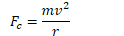# Uniform Circular Motion

• Object travels in a circular path at constant speed

• Distance around the circle is its circumference

•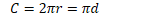• Average speed formula from kinematics still applies

•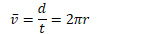# Frequency

• Frequency is the number of revolutions or cycles which occur each second

• Symbol is f

• Units are 1/s, or Hertz (Hz)

•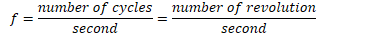# Period

• Period is the time it takes for one complete revolution, or cycle.

• Symbol is T

• Unites are seconds (s)

• T = time for 1 cycle = time for 1 revolution

# Frequency and Period

••# Centripetal Acceleration

• Is an object undergoing UCM accelerating?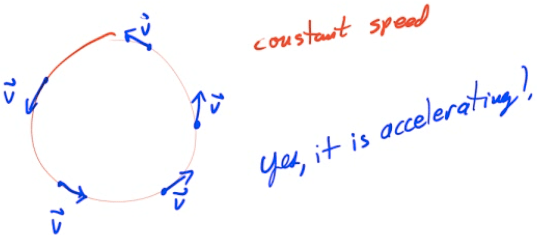• Magnitude of Centripetal Acceleration

•# Centripetal Force

• If an object is traveling in a circle it is accelerating toward the center of the circle

• For an object to accelerate, there must be a net force

••